# Further MechanicsMechanics Essential Notes (Part 2) Notes on lots of the further maths mechanics topics. See the other mechanics page for notes on single A level mmaths mechanics topics. PDF here.Notes on Moments A Level Mechanics course notes on 'Moments' (written for the 2017 New Spec). PDF here.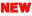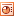Centre of Mass of a Glass of Water Which has the lower centre of mass out of an empty vs half-full vs full glass of water? How about the same question with a beaker instead? Or with a can, with a lid? PDF here.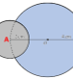Centre of Mass Practice Lots of practise in finding centre of mass. Can you find the centre of mass of each of these laminas? Includes answers.PDF here.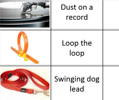Circular Motion Intro A good introductory task to considering circular motion. Students estimate radii, velocities and forces of everyday examples of circular motion. PDF here.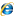Masses and Springs Lab A great external website demonstration of the conversions between GPE, KE and EPE and remaining constant as a whole.Vertical Circles A one page set of notes on circular motion and the energy forces involved in vertical circles. PDF here.